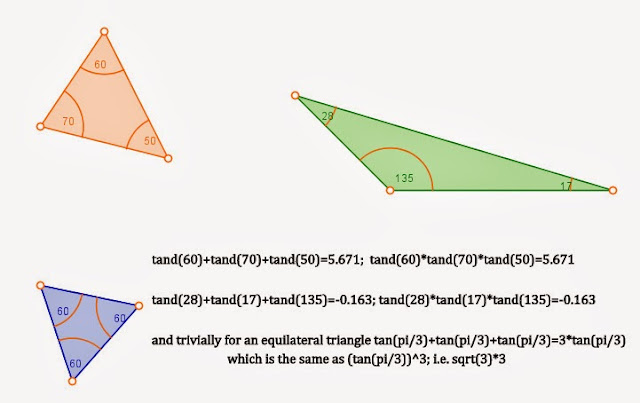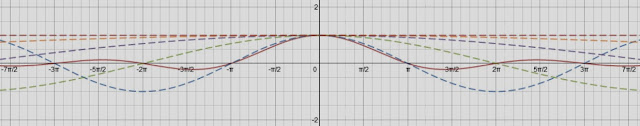## Idiosyncratic synesthetic experiences in some trivial trigonometric identities

Mathematical objects, despite existing in a purely abstract “Platonic” realm, have the ability (perhaps by the very virtue of their Platonic idealism) to produce synesthetic experiences. We have often wondered in our life as to why the realization or experience of such objects that do not belong to the real world produce such real even if very idiosyncratic experiences. Indeed, this synesthetic experience seems to form the fundament of what both layman and mathematician alike might term as beauty in mathematics [Footnote 1]. These need not stem from the experience of the understanding of extremely complex mathematical relationships; rather they can emerge from things as trivial as the experience of increasing dimensionality from a point, to a triangle to a tetrahedron. Not surprisingly, such mathematical objects often serve as metaphors (since metaphor is an expression of the synesthetic experience) in philosophy, religion and ritual of many cultures. In our own tradition such metaphors are abundant in many philosophical streams, like the decimal place value system being used in the sAMkhya of the vyAsa-bhAShya and by shaMkara the advaitin.

viShNu, on behalf of the fellow deva-s, during the pitched contest for the universe with the daitya-s, took three great strides. With these strides he won the whole universe for the deva-s and excluded the daitya-s from it. He is said to be viShNu because with this three strides he measured out or pervaded the whole universe – the old etymologists would say: “viShNur vishvaM vishate |”. We saw a paradox in this: The measuring out or the pervading of the universe is a volumetric action – i.e. you fill out the volume of the universe. But then the taking of strides has a sense of linearity to it: three steps would map as the summation of three lengths in a one dimensional space but how come a volume is implied as being filled?

Many, many revolutions of the earth ago, our father, in attempt to lead us to the path of mathematics, asked us to consider several triangles and take the sum of the tangents of the three angles of the triangle and see how they compared with their products. Being not very mathematically gifted, we set ourselves to the task in a slightly un-Hindu manner by drawing triangles and doing what we were asked to do:It amazed us to learn that given any triangle with angles a, b and c the following held true:
tan(a)+tan(b)+tan(c)=tan(a)*tan(b)*tan(c)

We saw immediately that it was rather trivial for an equilateral triangle or any right triangle or the extreme situation of a collapsed triangle (0,0, pi) but stopped at that. It also struck us that the value for the equilateral triangle (5.196) was the lowest positive value the that product or sum of the tangent triad took. We excitedly pointed this to our father. He seemed unimpressed and simply mumbled something and went to attend to his work.

Sometime later, armed with that wonderful elixir known as testosterone, when we teaching our brother trigonometry we effortlessly showed him why this beautiful relationship was rather trivial.
For a triangle:
a+b+c = pi;
a+b = pi-c;
tan(a+b) = tan(pi-c);
(tan(a)+tan(b))/(1-tan(a)tan(b)) =-tan(c);
thus: tan(a)+tan(b)+tan(c)=tan(a)*tan(b)*tan(c)
It then hit us that no wonder our father was unimpressed by our mental vapidity.

Of course, we showed more celerity when confronted by a problem based on this theorem in one of those life-deciding battles that come upon you in the realms of bhArata:
There is a result in algebra which says that arithmetic mean of n positive numbers is always greater than or equal to the geometric mean of the same. The tangents of the angles represent such a triad of numbers when the triangle is acute i.e.,is all angles are less than pi/2 as red triangle in the figure.
Hence, for such a triangle –
the arithmetic mean (tan(a)+tan(b)+tan(c))/3 >= (tan(a)*tan(b)*tan(c))^(1/3);
tan(a)*tan(b)*tan(c)/3 >= (tan(a)*tan(b)*tan(c))^(1/3);
(tan(a)*tan(b)*tan(c))^2 >= 27;
thus tan(a)*tan(b)*tan(c) >= 5.196 in an acute triangle. That lowest value is (tan(pi/3))^3, which is what we had stumbled upon in the exercise given to us in our childhood.

After the event as we were spilling out of the hall we remarked to our friends that it was rather trivial and went on (several of whom agreed since most of our circle were at the peak of our mathematical abilities at that point). But we also had a synesthetic experience that cut Gordian knot of the viShNu paradox: The product of 3 scalar entities is an abstraction of a volumetric mensuration. The sum of the same entities is like a linear measurement of 3 steps. Thus, we saw the equivalence between the 3 steps and a volumetric measurement. Moreover, that excluded region between 5.196 and 0 was the realm from which the daitya-s had been excluded.

We had at that point asked our student to show that it is obvious that:
sin(x)/x = ∏[n=1..∞]cos(x/2^n)
[
For those interested but unaware, this may be achieved thus, as was first shown by the great Leonhard Euler:
sin(x)/x= (sin(x/2)/(x/2))*cos(x/2) by half-angle formula for sin(x)
we do the same with sin(x/2) to get:
sin(x)/x= (sin(x/4)/(x/4))*cos(x/2)*cos(x/4)
We can continue thus ad infinitum:
Hence, we will have:
sin(x)/x= (sin(x/2^n)/(x/2^n))*∏[n=1..∞]cos(x/2^n)
but as n->∞, x/2^n->0, therefore sin(x/2^n)/(x/2^n)->1
thus, sin(x)/x = ∏[n=1..∞]cos(x/2^n)
]

The synesthetic experience of this relationship was both calming and foreboding (as in the sense of the end of existence): one of perturbations caused by an upheaval being reabsorbed by enormous magnitude of the unchanging fabric of existence (we felt a certain connection the sAMkhya term for the same: mahat – the enormity).
Imagine the function y=x/x it is the epitome of constancy, an unperturbed straight line – the metaphor of the unchanging fabric of existence. Now a perturbation emerges in the numerator in the form of a sine wave. Its normalization by the denominator makes that perturbation to fade out into nothingness, the y=0 straight line (like the sAMkhya universe fading out into mahat). The same thing as the product of increasingly halved cosines explicitly illustrates this, as those halved cosines come closer and closer to our original line y=x/x.-۩۞۩-۩۞۩-۩۞۩-
Footnote 1: Some mathematicians find something they call rigor to be the basis for the beauty. We had a mathematics teacher who loved using the word rigor ad nauseum. But to us rigor was never a major source of inspiration or interest – for we simply saw gaNita as either a generator of beauty or a tool for vyavahAra in the real world.

This entry was posted in art, Heathen thought, Life and tagged , , , , , , , . Bookmark the permalink.